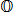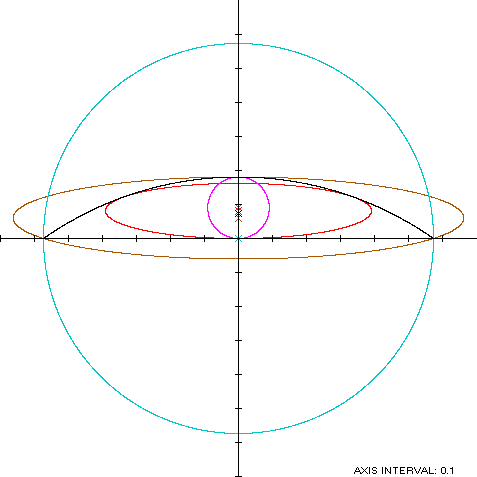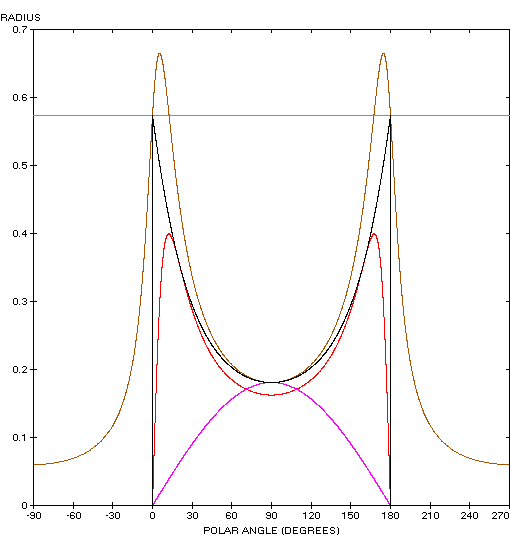Circular Segment
CIRCULAR SEGMENT

Balmoral Software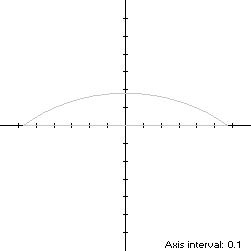Let a circular segment S of radius 1 be oriented with the origin at the midpoint of the chord connecting the endpoints of its arc, and with the center of the circle on the negative y-axis, so that S is symmetric with respect to that axis. If β < π/2 is one-half the angle subtended by the segment arc, then the extreme abscissa points of S are (±sin(β),0), the maximum ordinate point is (0,1 - cos(β)), and the width x height of the bounding rectangle of S is 2sin(β) x 1 - cos(β).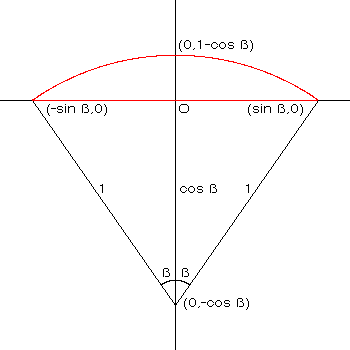Let t represent the usual parameter, ranging from 0 to 2π through the quadrants in counterclockwise order. In Quadrants I and II, the parametric equations of the segment arc are:
x(φ) = cos(φ)

y(φ) = sin(φ) - cos(β),

where the angle φ is measured from the center of the circle and ranges from π/2 - β to π/2 + β. We can scale t to the angular parameter φ as follows:
 Range of t Range of φ [0,π] [π/2 - β,π/2 + β]
or equivalently,
φ = 2βt/π + π/2 - β
In Quadrants III and IV, the parametric function x(t) of the horizontal chord is linear in t. Combining definitions, we have### Metrics

The perimeter of the segment is the arc length of the circular arc (in radians) plus the chord:
L = 2β + 2sin(β)
The area of S is
A = [2β - sin(2β)]/2
and its centroid ordinate is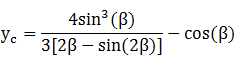Specific Example

In the remainder of this paper, we use

β = 35° = 7π/36

L = 2.368883
A = 0.141019
yc = 0.072931
Bounding rectangle: 1.147153 x 0.180848

### Incircle

A candidate incircle is located on the y-axis between the ordinate extrema of S, and so has radius and center ordinate both equal to
R = d = [1 - cos(β)]/2 = 0.090424
For verification, we have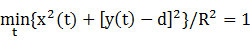### Inellipse

Using z = 0 in Lemma E,
d/dt x(t)[y(t) - z] = d/dt cos(2βt/π + π/2 - β)[sin(2βt/π + π/2 - β) - cos(β)]
has a zero over [0,π) at t* = 0.682719. The corresponding coordinates are
x* = 0.338539

y* = 0.121800

We then have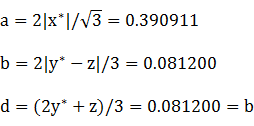For verification,### Circumellipse

The extreme points of the segment create a y-symmetric isosceles triangle with vertices (0,1 - cos(β)) and (±sin(β),0). Using h = 1 - cos(β) and w = 2sin(β) in Lemma TE, a candidate for the circumcircle has parameters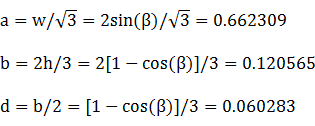For verification,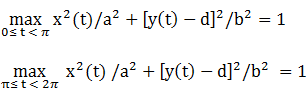### Circumcircle

The smallest enclosing circle is one with the chord of S as its diameter, so
R = sin(β) = 0.573576

d = 0

Since the radius of the circumcircle is less than the unit radius of the segment, the circumcircle has greater curvature and thus encloses S.

### Summary Table

 Perimeter Area Centroid Figure Parameters Incircle R = [1 - cos(β)]/2 0.568151 0.025687 (0,0.090424) Inellipse a = 0.390911b = 0.081200 1.649400 0.099720 (0,0.081200) Circular segment Width: 2sin(β)Height: 1 - cos(β) 2.368883 0.141019 (0,0.072931) Circumellipse a =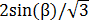b =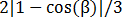2.765929 0.250860 (0,0.060283) Circumcircle R = sin(β) 3.603887 1.033552